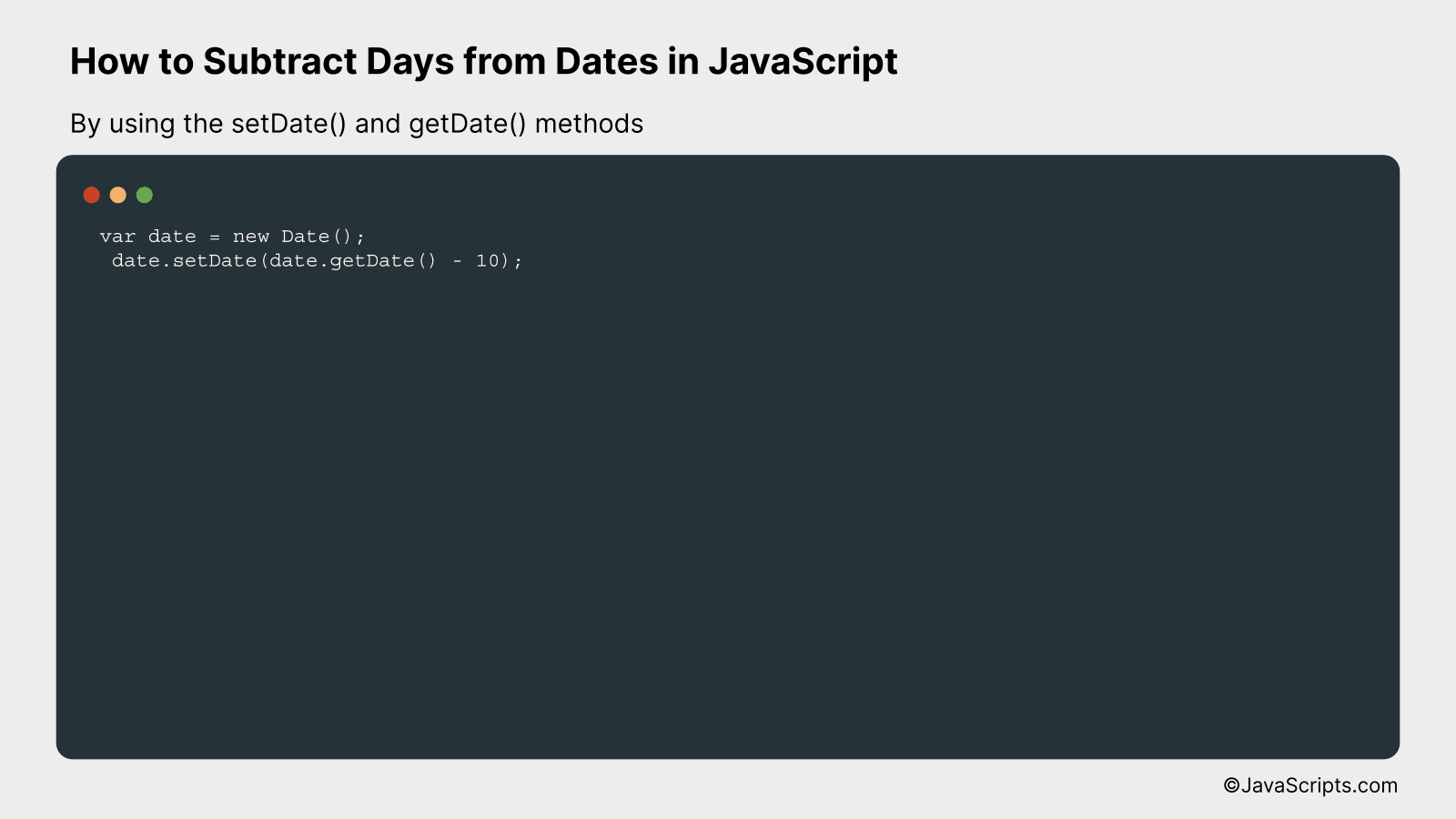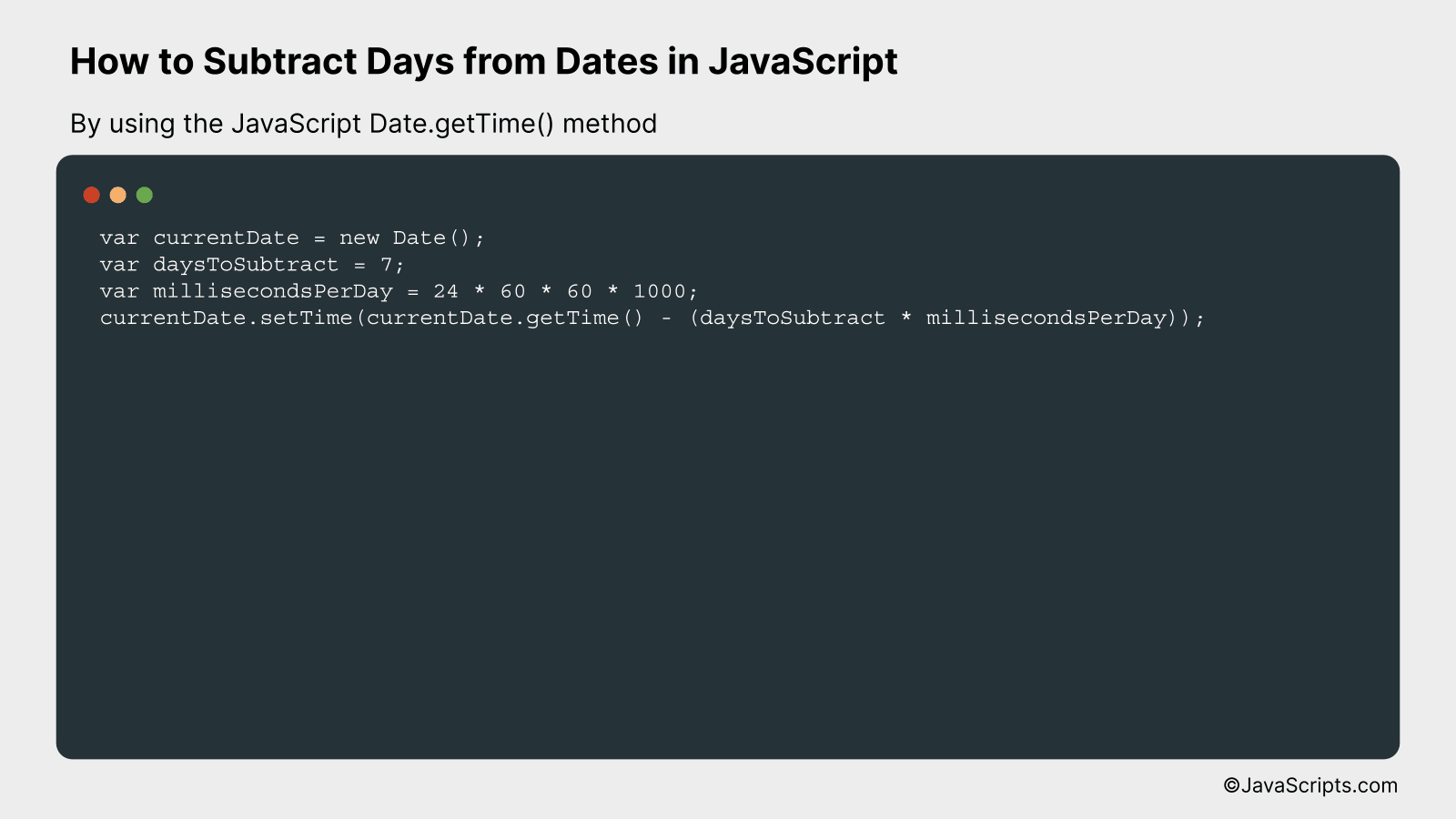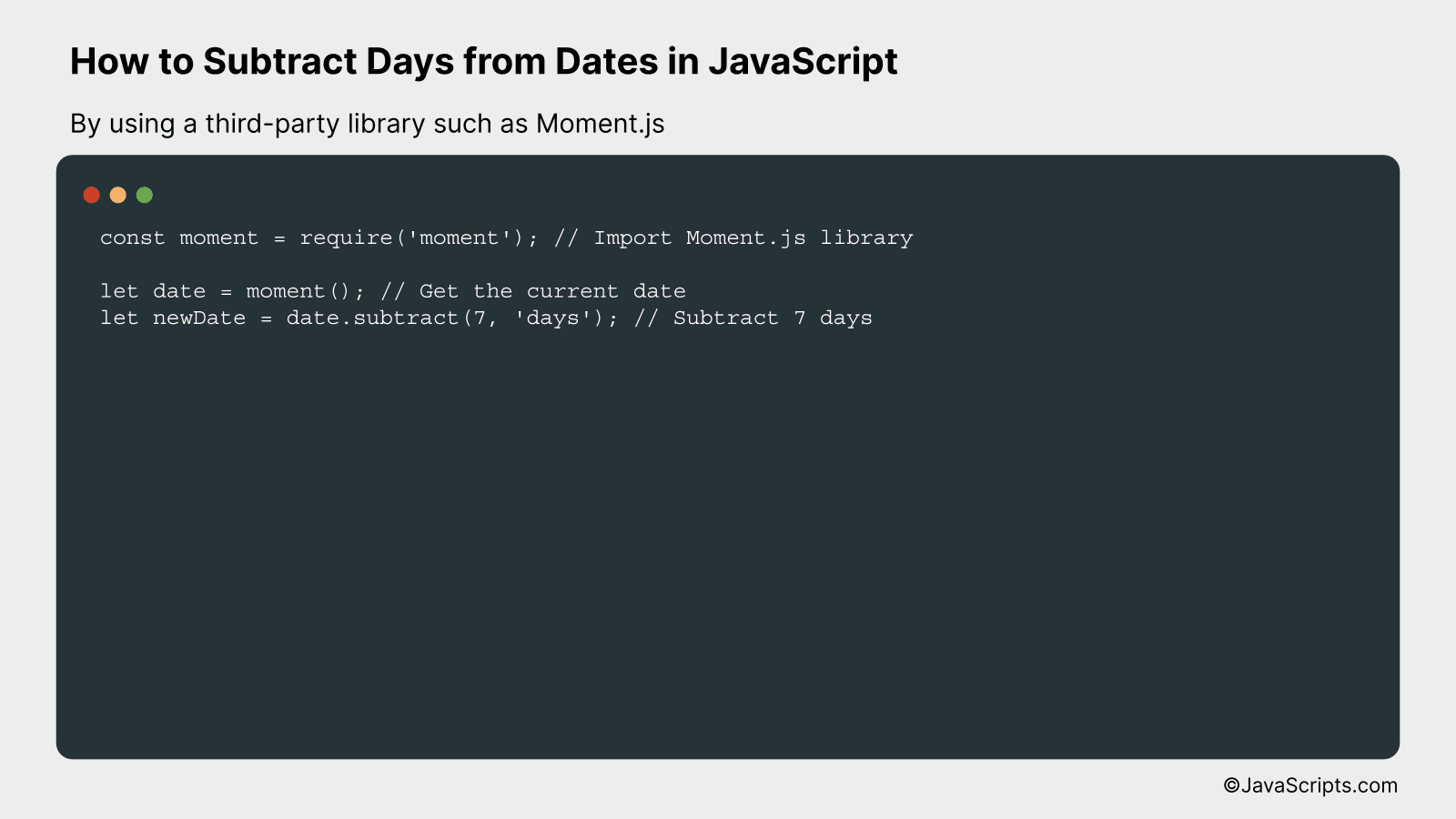# How to Subtract Days from Dates in JavaScript

Have you ever been working on a JavaScript project and found yourself needing to subtract days from dates? You’re not alone! This is a common task in many web applications, especially those dealing with scheduling, booking, or data tracking.

Don’t worry if you’re not sure how to approach this problem. We’re about to dive into a straightforward and easy-to-understand method to do just that. With a touch of JavaScript magic, you’ll be manipulating dates like a pro in no time.

In this post, we will be looking at the following 3 ways to subtract days from dates in JavaScript:

• By using the setDate() and getDate() methods
• By using the JavaScript Date.getTime() method
• By using a third-party library such as Moment.js

Let’s explore each…

## #1 – By using the setDate() and getDate() methodsIn JavaScript, you can subtract days from a date by using the setDate() and getDate() methods. This works by getting the current day of the month with getDate(), subtracting the number of days you want, and then updating the date with setDate(). For instance, subtracting 10 days from the current date would look like this:

``````
var date = new Date();
date.setDate(date.getDate() - 10);
``````

### How it works

This code first creates a new Date object, which defaults to the current date and time. It then gets the current day of the month (a number between 1 and 31) with getDate(). After that, it subtracts the specified number of days from this value. Finally, it updates the date to this new day of the month with setDate().

• Step 1: The `var date = new Date();` creates a new Date object with the current date and time.
• Step 2: `date.getDate()` is used to get the current day of the month (a number from 1 to 31).
• Step 3: We subtract the desired number of days from the current day of the month. In the example, we subtract 10: `date.getDate() - 10`.
• Step 4: We then set the date of the “date” object to this new value with `date.setDate()`. This effectively subtracts the desired number of days from the original date.

## #2 – By using the JavaScript Date.getTime() methodSubtracting days from a date in JavaScript can be achieved by using the JavaScript Date.getTime() method. This method returns the number of milliseconds since the Unix Epoch (January 1, 1970 00:00:00 UTC). By subtracting the desired number of days (converted to milliseconds) from the current date, you can get a new date corresponding to the desired past date. For instance, if you want to find the date 7 days before today, you will subtract the milliseconds equivalent of 7 days from today’s date.

Here’s the code example:

``````
var currentDate = new Date();
var daysToSubtract = 7;
var millisecondsPerDay = 24 * 60 * 60 * 1000;
currentDate.setTime(currentDate.getTime() - (daysToSubtract * millisecondsPerDay));
```
```

### How it works

The code above works by first getting the current date and time using Date() constructor which is then stored in the variable ‘currentDate’. The number of days you want to subtract is stored in the variable ‘daysToSubtract’.

We know that there are 24 hours in a day, 60 minutes in an hour, 60 seconds in a minute, and 1000 milliseconds in a second. So, we calculate the total number of milliseconds in a day and store it in the ‘millisecondsPerDay’ variable. Then, we get the number of milliseconds for the current date and time using the getTime() method. From this, we subtract the product of ‘daysToSubtract’ and ‘millisecondsPerDay’ to get the new date and time, which is then set as the new value for ‘currentDate’.

Here’s a step-by-step explanation:

1. Create a new Date object ‘currentDate’ that holds the current date and time.
2. Define the number of days you want to subtract and store it in the ‘daysToSubtract’ variable.
3. Calculate the number of milliseconds in a day and store it in the ‘millisecondsPerDay’ variable.
4. Use the getTime() method on ‘currentDate’ to get the number of milliseconds since Unix Epoch for the current date and time.
5. Subtract the product of ‘daysToSubtract’ and ‘millisecondsPerDay’ from the number of milliseconds since Unix Epoch for the current date and time.
6. Finally, set this new value to ‘currentDate’ using the setTime() method. Now, ‘currentDate’ represents the date and time that is ‘daysToSubtract’ days before the original date and time.

## #3 – By using a third-party library such as Moment.jsThis process involves subtracting a certain number of days from a given date by utilizing Moment.js, a robust JavaScript library for parsing, validating, manipulating, and formatting dates. We’ll walk through an example where we subtract 7 days from the current date.

``````
const moment = require('moment'); // Import Moment.js library

let date = moment(); // Get the current date
let newDate = date.subtract(7, 'days'); // Subtract 7 days
``````

### How it works

The code snippet uses Moment.js to perform date arithmetic – specifically, subtracting days from a specified date. It first gets the current date and then subtracts 7 days from it.

• First, we import the Moment.js library using `require('moment')`.
• Next, we create a Moment.js date object for the current date with `moment()`. The resulting object represents the current date and time.
• Then, we use the `subtract` function provided by Moment.js to subtract a specified number of days (in this case, 7) from the given date.
• The `subtract` function manipulates the original Moment.js date object, effectively changing the date.
• The result is a new date object representing the date 7 days prior to the current date.

Related:

In closing, mastering date manipulation in JavaScript is a valuable skill that can make your work more efficient and precise. The process of subtracting days from dates may seem complex at first, but with practice, it becomes straightforward.

Remember, the Date object is your friend in this, coupled with the setDate() and getDate() methods. Don’t get intimidated, instead, keep experimenting and learning. It’s all about practice and patience to get a good grip on any coding technique.